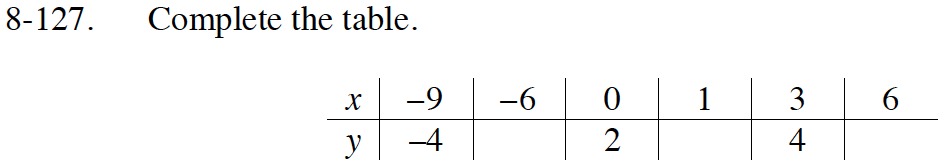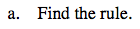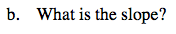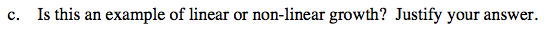Home > ACC7 > Chapter cc38 > Lesson cc38.3.1 > Problem8-127

8-127.
1. Complete the table. 8-127 HW eTool (Desmos). Homework Help ✎

 x –9 –6 3 0 1 6 y –4 4 2
1. Find the rule.

2. What is the slope?

3. Is this an example of linear or non-linear growth? Justify your answer.Notice that there are three known coordinates: (−9, −4), (0, 2), and (3, 4).

Now notice that for every difference of 3 in the
x-coordinate, the y-coordinate changes by 2.
Use this reasoning to fill in the rest of the table.

The difference in the y-coordinate from x = 0 to x = 3 is 2. Since 6 is twice 3, the difference in the y-coordinate from x = 0 to x = 6 is twice as much.

(−6, −2) and (6, 6)To find the rule, look for a pattern that can relate the
y-coordinate to the x-coordinate.

Notice that at x = 0, y = 2.
Also remember that the y-coordinate increases by 2 for every 3 units the x-coordinate increases.The slope describes how the values increase.
It can be written as:

$\frac{\text{change in }{\it y}}{\text{change in }{\it x}}$

${\it y} = \frac{2}{3}{\it x} + 2$

You can fill in the last y-coordinate in the table by substituting 1 into the rule.If you were to graph all of the points in the table, would they form a line?

Complete the table in the eTool below to graph the points.
Click the link at right for the full version of the eTool: CC3 8-127 HW eTool